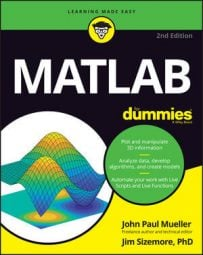##### MATLAB For DummiesMATLAB is used in a lot of different ways by lots of people in occupations you might not necessarily think about when it comes to a math product. In fact, math is used in ways that many people don’t consider.

## Engineering new solutions

Many engineering disciplines rely on various kinds of math to ensure that the results of any design process or new theory of how the universe works actually make sense. A new building isn’t much use if it can’t hold up to the stresses placed on it.

Creating new technologies means first computing how this new technology will work. Many ideas and concepts used in engineering rely on math as a starting point because math provides the means to express the idea in a form that others can understand.

## Getting an education

Mathworks places a strong emphasis on education. In fact, you can find a special place for education-related materials at MathWorks.com. Even if the organization that employs you doesn’t use MATLAB, the principles you learn by working through problems with MATLAB follow standards that apply equally well to other products.

One of the more useful resources that you find on the MATLAB site is the list of tutorials.

If you’re a student and you need a copy of MATLAB, you can easily purchase it. Several versions of MATLAB are available for student use, so make sure that you pick the package that best suits your needs. Note that you may require some special add-ons for your classes.

## Working with linear algebra

It may be hard to believe, but linear algebra really is part of the workplace. For example, to calculate Return on Investment (ROI), you must know algebra. The same holds true for the following:

• Predicting the amount of turnover a company will have

• Determining how many items to keep in inventory

• Making life and business decisions, such as whether it’s cheaper to rent a car or to buy one outright

• Creating a financial plan, such a determining whether it makes more sense to pay down a credit card or build up savings

If your job is helping people perform these sorts of tasks, you really do need something like MATLAB so that you can get the answers you need fast.

## Performing numerical analysis

Numerical analysis relies on approximation rather than the precision you see in symbolic math. Performing certain building construction tasks is impossible without applying numerical analysis, and astronomy seems to require heavy use of it as well. You probably won’t see a carpenter applying numerical analysis, but you will see architects who might need to do so.

## Getting involved in science

MATLAB is likely to be used to explore new theories. When applied to science, MATLAB helps you perform “what if” analysis that helps you confirm the viability of a theory.

Of course, science is used in many different ways. For example, you might be involved in the health industry and using science to find a cure for cancer or the Ebola virus. A computer scientist might look for a new way to use computer technology to aid those with accessibility needs.

## Engaging mathematics

Some people simply enjoy playing with math. It’s the reason that so many theorems are available today to solve problems. These people are engaged with math in a way that few others can readily understand. MATLAB makes it possible to play with math, to create new ways of using numbers to perform useful tasks.

## Exploring research

After a question is asked and an answer is given, a researcher must convince colleagues that the answer is correct and then viable to put into practice. MATLAB lets you check the answer and verify that it does, in fact, work as the researcher suggests. After an answer is proven, the researcher can use MATLAB further to define precisely how the answer is used.

Although many of MATLAB’s uses require graphics, research has a significant need for graphics because the researcher must often explain answers to people who don’t have the researcher’s skills.

## Walking through a simulation

Using a simulation rather than a real-world counterpart is a low-cost approach to testing that is an essential part of any sort of scientific or engineering endeavor today, for these reasons:

• Saves human lives

• Saves time

• Enhances the ability of the people involved to try various solutions

• Reduces costs

• Improves the chances of a new technology succeeding

• Increases the security surrounding a new technology

MATLAB makes simulations possible in several different ways. It may not always provide a complete solution, but you can use it to perform these kinds of tasks:

• Define the original math model used to define the technology and therefore the simulation

• Create individual snapshots showing how the technology will work based on the model

• Demonstrate the workflow for a technology using animation techniques so that even less-skilled stakeholders can see the technology at work

## Employing image processing

Image processing is the act of managing the pixels in an image using math techniques to modify the matrix values. Techniques such as adding two matrices together are common when performing image processing.

The one thing you should know is that MATLAB excels at matrix manipulation. Anyone involved in image processing needs the sort of help that MATLAB provides to create and test new image processing techniques.

## Embracing programming using computer science

Computer scientists rely heavily on math to perform tasks. MATLAB, with its rich toolbox, can be used to rapidly prototype an algorithm before committing the development resources to implementing the algorithm in another language, such as C++ or Java. Programmers commonly depend on MATLAB to enhance their productivity.

When creating an application, you must ensure that the output is valid. However, verification is just one use. Computer scientists deal with a nearly inexhaustible supply of unknowns that could potentially benefit from the use of MATLAB.